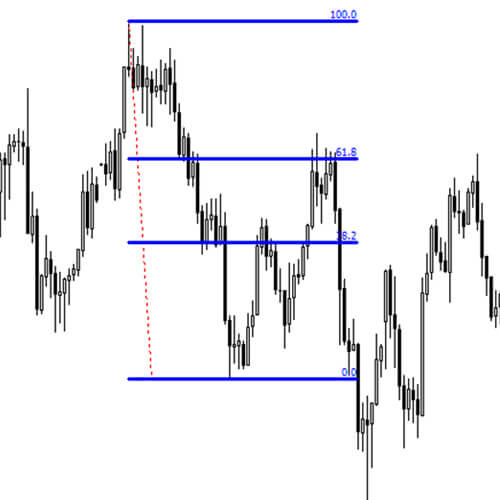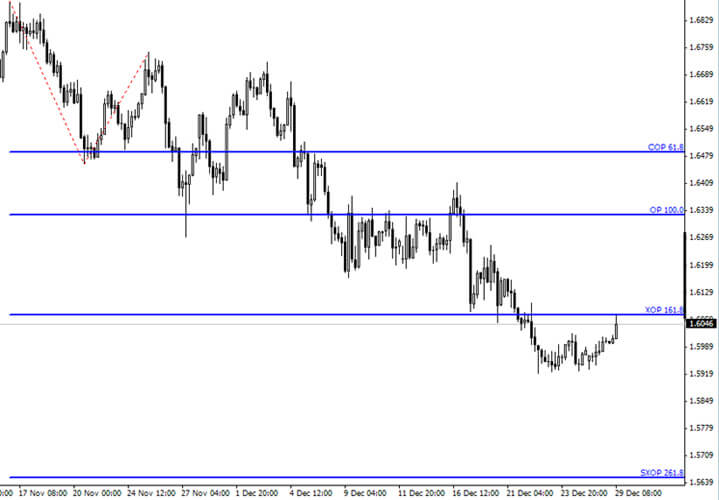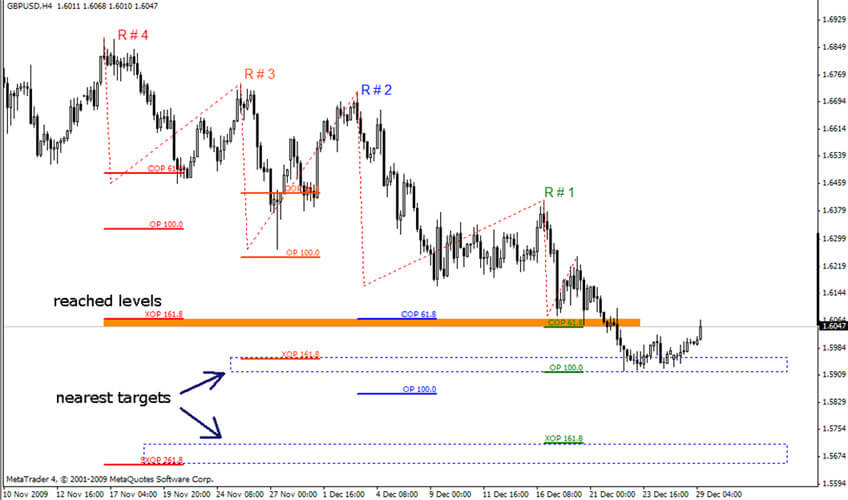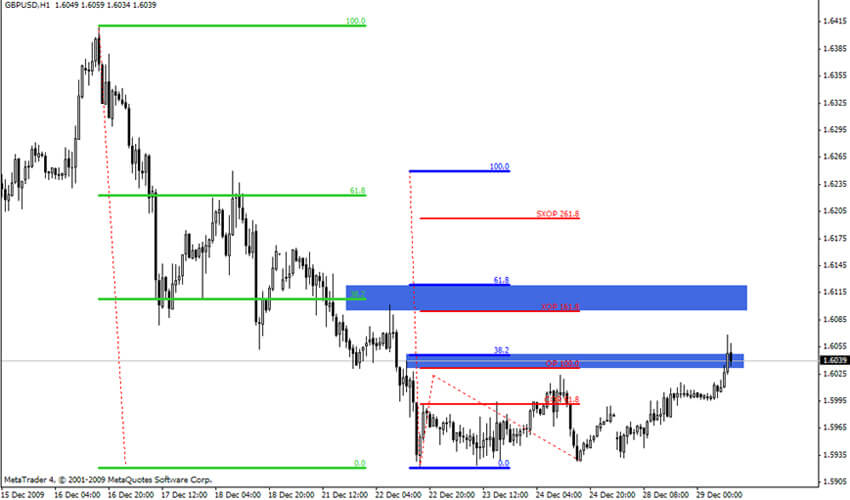# HOW TO USE FIBONACCI LEVELS IN DINAPOLI STYLE

No comment 1091 views
5 / 5 ( 1votes )

Below is a summary of how to use the Fibonacci level when trading in the DiNapoli style.

Of all retracement levels available (.236, .382, .50, .618, .764) J. In Napoli only choose 2 – 382 and 618. which one you enter at -61.8% or 76.4% – in the case that both protective stops will be below / above the start of the wave (reaction). You can see how corrective fib-nodes are seen in Fig. 1. And knowing the initial two DiNapoli techniques is more than enough.

Figure 1. Repair of fib-nodesThe number of expansion levels is not too large, the author uses 3 levels and one more – a highly expanded objective point – added by other traders using the technique.

1. COP – contracted objective point = 618 of wave A measured from the end of the corrective wave B;

2. ОР – objective point = 100% of the length of wave A measured from the end of wave B;

3. XOP – expanded objective point = 1.618 of wave A measured from B;

4. SXOP – super expanded objective point = 2.618 of wave A measured from B.

The most interesting and logical point in applying this particular extension is that the length of the corrective wave also plays a role – all measurements start from the end of the corrective wave B. I believe this is a very logical and reasonable technique.Neophytes for currency trading often calculate the extension and retracement of wave roles and places that are somewhat understood. To sort out some of Joe DiNapoli’s calculations introducing the term “reaction number” that gives specific fib-node repair. The following is a screenshot showing the reaction number and each Fibonacci extension. Thanks to the reaction number you will know what each expansion point means and where it comes from. You can also see the “dating” area – where the expansions off the various reaction numbers fall into at least about one area.The last screenshot shows all actual, expanding and Fibonacci retracements where they cross each other, which provides strong support and resistance.Forex Signal 30 is the best forex system since 2009 and has been used by thousands of traders from around the world to generate profit in forex trading. This system is created by our team of Brilliant Forex Signal Team, this system is made as simple as possible for beginner and professional traders.